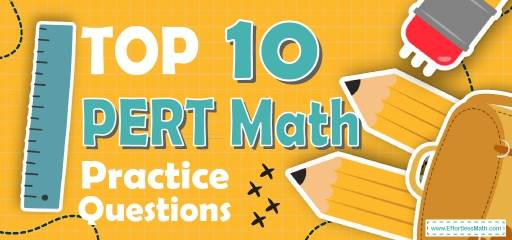# Top 10 PERT Math Practice QuestionsTaking the PERT Math Test in a few weeks or even in a few days? The best way to prepare for your PERT Math test is to work through as many PERT Math practice questions as possible. Here are the top 10 PERT Math practice questions to help you review the most important PERT Math concepts. These PERT Math practice questions are designed to cover mathematics concepts and topics that are found on the actual test. The questions have been fully updated to reflect the latest 2022 PERT guidelines. Answers and full explanations are provided at the end of the post.

Start your PERT Math test prep journey right now with these sample PERT Math questions.

## PERT Math Practice Questions

1- $$(x + 7) (x + 5) =$$ ?

A.$$x^2 + 12x + 12$$

B. $$2x + 12x + 12$$

C. $$x^2 + 35x + 12$$

D. $$x^2 + 12x + 35$$

2- If $$x$$ is a positive integer divisible by 6, and $$x < 60$$, what is the greatest possible value of $$x$$?

A. $$54$$

B. $$48$$

C. $$36$$

D. $$59$$

3- $$x^2 – 81 = 0$$, $$x$$ could equal to:

A. $$6$$

B. $$9$$

C. $$12$$

D. $$15$$

4- If $$a = 8$$, what is the value of $$b$$ in this equation?
$$b=\frac{a^2}{4}+4$$

A. $$24$$

B. $$22$$

C. $$20$$

D. $$28$$

5- If $$6.5 < x ≤ 9.0$$, then $$x$$ cannot be equal to:

A. $$6.5$$

B. $$9$$

C. $$7.2$$

D. $$7.5$$

6- What is the area of an isosceles right triangle that has one leg that measures $$6 cm$$?

A. $$18 cm$$

B. $$36 cm$$

C. $$6 \sqrt{2}$$ $$cm$$

D. $$72 cm$$

7- Which of the following expressions is equivalent to $$10 – \frac{2}{3} x ≥ 12$$

A. $$x ≥ –3$$

B. $$x ≤ –3$$

C. $$x ≥ 24 \frac{1}{3}$$

D. $$x ≤24 \frac{1}{3}$$

8- Which of the following is a factor of both $$x^2 – 2x – 8$$ and $$x^2 – 6x + 8$$?

A. $$(x – 4)$$

B. $$(x +4)$$

C. $$(x – 2)$$

D. $$(x +2)$$

9- $$\frac{1}{6b^2} + \frac{1}{6b} = \frac{1}{b^2}$$, then $$b =$$?

A. $$-\frac{16}{15}$$

B. $$5$$

C. $$-\frac{15}{16}$$

D. $$8$$

10- If two angles in a triangle measure $$53$$ degrees and $$45$$ degrees, what is the value of the third angle?

A. $$8$$ degrees

B. $$42$$ degrees

C. $$82$$ degrees

D. $$98$$ degrees

## Best PERT Math Prep Resource for 2022

1- D
Use FOIL (First, Out, In, Last)
$$(x + 7) (x + 5) = x^2 + 5x + 7x + 35 = x^2 + 12x + 35$$

2- A
$$\frac{54}{6}=\frac{27}{3}=9$$
$$\frac{48}{6}=\frac{24}{3}= 8$$
$$\frac{36}{6}=\frac{18}{3}=6$$
$$\frac{59}{6}=\frac{59}{6}$$
$$59$$ is prime number

3- B
$$x^2 – 81 = 0 ⇒ x^2 = 81 ⇒ x = 9$$

4- C
If $$a = 8$$ then $$b =\frac{8^2}{4} + 4$$ ⇒ $$b = \frac{64}{4} + 4 ⇒ b = 16 + 4 = 20$$

5- A
If $$6.5 < x ≤ 9.0$$, then $$x$$ cannot be equal to $$6.5$$.

6- A
$$a=6$$⇒ area of triangle is $$=\frac{1}{2} (6×6)=\frac{36}{2}=18 cm$$

7- B
Simplify:
$$10 – \frac{2}{3} x ≥ 12 ⇒ – \frac{2}{3}2 x ≥ 2 ⇒ – x ≥ 3 ⇒ x ≤ – 3$$

8- A
Factor each trinomial $$x^2 – 2x – 8$$ and $$x^2 – 6x + 8$$
$$x^2 – 2x – 8 ⇒ (x – 4)(x + 2)$$
$$x^2 – 6x + 8 ⇒ (x – 2)(x – 4)$$

9- B
$$\frac{1+b}{6b^2}=\frac{1}{b^2}$$
$$⇒(b≠0) b^2+b^3=6b^2⇒b^3-5b^2=0⇒b^2 (b-5)=0⇒b-5=0⇒b=5$$

10- C
$$53^\circ + 45^\circ= 98^\circ$$
$$180^\circ– 98^\circ= 82^\circ$$
The value of the third angle is $$82^\circ$$.

Looking for the best resource to help you succeed on the PERT Math test?

## More from Effortless Math for PERT Test …

### Are you looking for PERT math prep books that have all the special features together?

Top 10 PERT Math Prep Books (Our 2022 Favorite Picks) will help you find such a book.

### Want to know how the math section of the PERT test is organized?

Overview of the PERT Mathematics Test provides the information you need.

### Do you need a lot of practice to master the PERT math test?

PERT Math Worksheets: FREE & Printable takes you to the mastery level.

## Have any questions about the PERT Test?

### What people say about "Top 10 PERT Math Practice Questions - Effortless Math: We Help Students Learn to LOVE Mathematics"?

No one replied yet.

X
49% OFF

Limited time only!

Save Over 49%

SAVE $34 It was$69.99 now it is \$35.99